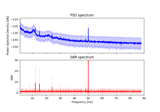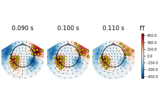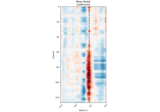# mne.viz.plot_topomap#

mne.viz.plot_topomap(data, pos, vmin=None, vmax=None, cmap=None, sensors=True, res=64, axes=None, names=None, show_names=False, mask=None, mask_params=None, outlines='head', contours=6, image_interp='bilinear', show=True, onselect=None, extrapolate='auto', sphere=None, border='mean', ch_type='eeg', cnorm=None)[source]#

Plot a topographic map as image.

Parameters
data`array`, shape (n_chan,)

The data values to plot.

pos`array`, shape (n_chan, 2) | instance of `Info`

Location information for the data points(/channels). If an array, for each data point, the x and y coordinates. If an `Info` object, it must contain only one data type and exactly `len(data)` data channels, and the x/y coordinates will be inferred from the montage applied to the `Info` object.

vmin

The value specifying the lower bound of the color range. If None, and vmax is None, the opposite of the maximum absolute value `-max(abs(data))` is used. Else `min(data)` is used. If callable, the output equals `vmin(data)`. Defaults to None.

vmax

The value specifying the upper bound of the color range. If None, the maximum value is used. If callable, the output equals `vmax(data)`. Defaults to None.

cmapmatplotlib colormap | `None`

Colormap to use. If None, `'Reds'` is used for all positive data, otherwise defaults to `'RdBu_r'`.

sensors

Add markers for sensor locations to the plot. Accepts matplotlib plot format string (e.g., `'r+'` for red plusses). If True (default), circles will be used.

res`int`

The resolution of the topomap image (number of pixels along each side).

axesinstance of `Axes` | `None`

The axes to plot to. If None, the current axes will be used.

names

List of channel names. If None, channel names are not plotted.

show_names

If True, show channel names on top of the map. If a callable is passed, channel names will be formatted using the callable; e.g., to delete the prefix ‘MEG ‘ from all channel names, pass the function `lambda x: x.replace('MEG ', '')`. If `mask` is not None, only significant sensors will be shown. If `True`, a list of names must be provided (see `names` keyword).

mask`ndarray` of bool, shape (n_channels,) | `None`

Array indicating channel(s) to highlight with a distinct plotting style. Array elements set to `True` will be plotted with the parameters given in `mask_params`. Defaults to `None`, equivalent to an array of all `False` elements.

Additional plotting parameters for plotting significant sensors. Default (None) equals:

```dict(marker='o', markerfacecolor='w', markeredgecolor='k',
linewidth=0, markersize=4)
```
outlines‘head’ | ‘skirt’ | `dict` | `None`

The outlines to be drawn. If ‘head’, the default head scheme will be drawn. If ‘skirt’ the head scheme will be drawn, but sensors are allowed to be plotted outside of the head circle. If dict, each key refers to a tuple of x and y positions, the values in ‘mask_pos’ will serve as image mask. Alternatively, a matplotlib patch object can be passed for advanced masking options, either directly or as a function that returns patches (required for multi-axis plots). If None, nothing will be drawn. Defaults to ‘head’.

contours

The number of contour lines to draw. If `0`, no contours will be drawn. If an array, the values represent the levels for the contours. The values are in µV for EEG, fT for magnetometers and fT/m for gradiometers. Defaults to `6`.

image_interp`str`

The image interpolation to be used. All matplotlib options are accepted.

showbool

Show figure if True.

onselect

Handle for a function that is called when the user selects a set of channels by rectangle selection (matplotlib `RectangleSelector`). If None interactive selection is disabled. Defaults to None.

extrapolate`str`

Options:

• `'box'`

Extrapolate to four points placed to form a square encompassing all data points, where each side of the square is three times the range of the data in the respective dimension.

• `'local'` (default for MEG sensors)

Extrapolate only to nearby points (approximately to points closer than median inter-electrode distance). This will also set the mask to be polygonal based on the convex hull of the sensors.

• `'head'` (default for non-MEG sensors)

Extrapolate out to the edges of the clipping circle. This will be on the head circle when the sensors are contained within the head circle, but it can extend beyond the head when sensors are plotted outside the head circle.

Changed in version 0.21:

• The default was changed to `'local'` for MEG sensors.

• `'local'` was changed to use a convex hull mask

• `'head'` was changed to extrapolate out to the clipping circle.

New in version 0.18.

sphere`float` | array-like | instance of `ConductorModel`

The sphere parameters to use for the cartoon head. Can be array-like of shape (4,) to give the X/Y/Z origin and radius in meters, or a single float to give the radius (origin assumed 0, 0, 0). Can also be a spherical ConductorModel, which will use the origin and radius. Can also be None (default) which is an alias for 0.095. Currently the head radius does not affect plotting.

New in version 0.20.

border`float` | ‘mean’

Value to extrapolate to on the topomap borders. If `'mean'` (default), then each extrapolated point has the average value of its neighbours.

New in version 0.20.

ch_type`str`

The channel type being plotted. Determines the `'auto'` extrapolation mode.

New in version 0.21.

cnorm

Colormap normalization, default None means linear normalization. If not None, `vmin` and `vmax` arguments are ignored. See Notes for more details.

New in version 0.24.

Returns
im`matplotlib.image.AxesImage`

The interpolated data.

cn`matplotlib.contour.ContourSet`

The fieldlines.

Notes

The `cnorm` parameter can be used to implement custom colormap normalization. By default, a linear mapping from vmin to vmax is used, which correspond to the first and last colors in the colormap. This might be undesired when vmin and vmax are not symmetrical around zero (or a value that can be interpreted as some midpoint). For example, assume we want to use the RdBu colormap (red to white to blue) for values ranging from -1 to 3, and 0 should be white. However, white corresponds to the midpoint in the data by default, i.e. 1. Therefore, we use the following colormap normalization `cnorm` and pass it as the the `cnorm` argument:

```from matplotlib.colors import TwoSlopeNorm
cnorm = TwoSlopeNorm(vmin=-1, vcenter=0, vmax=3)
```

Note that because we define `vmin` and `vmax` in the normalization, arguments `vmin` and `vmax` to `plot_topomap` will be ignored if a normalization is provided. See the matplotlib docs for more details on colormap normalization.

## Examples using `mne.viz.plot_topomap`#Frequency-tagging: Basic analysis of an SSVEP/vSSR dataset

Frequency-tagging: Basic analysis of an SSVEP/vSSR datasetHow to plot topomaps the way EEGLAB does

How to plot topomaps the way EEGLAB doesPlotting topographic maps of evoked data

Plotting topographic maps of evoked dataReceptive Field Estimation and Prediction

Receptive Field Estimation and Prediction